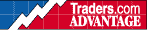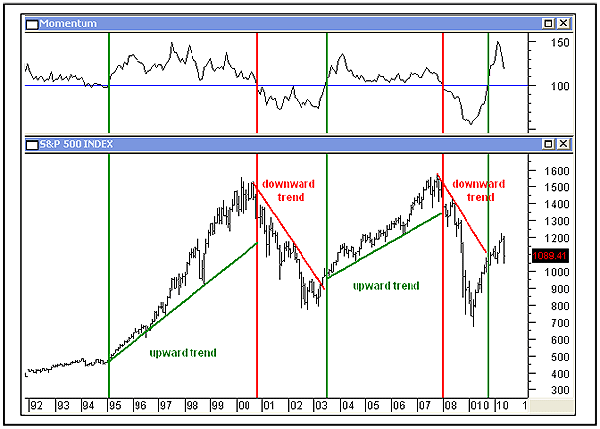HOT TOPICS LIST

INDICATORS LIST

LIST OF TOPICS

# Momentum Indicator Measures Trend

06/29/10 09:36:47 AM
by Alan R. Northam

When we are using a momentum indicator, it is important to know what is being analyzed.

Security:   SPX
Position:   N/A

 There are many indicators that can measure momentum. Among these are the rate of change (ROC), the relative strength (RS), the stochastic, the moving average convergence/divergence (MACD), and the momentum indicator, among others. This article deals with the momentum indicator. The momentum indicator is plotted by dividing the closing price of so many periods ago into the most recent closing price. An example would be to divide the closing price of a stock exactly six months ago into today's closing price. The close of six months ago is called the lookback period. The result of this division is a ratio between the most recent price and the price at the lookback period. This result is known as a ratio and is plotted on the chart. If the most recent closing price and the closing price of the lookback period, say six months ago, are the same, then the ratio is equal to 1. The ratio is then multiplied by 100 to put it in percentage and plotted on the chart as 100%. If the most recent price is higher than the price at the lookback period, that ratio is multiplied by 100 and plotted on the chart at a value above 100%. If the most recent closing price is less than the close at the lookback, the ratio becomes less than 1. Again, this ratio is multiplied by 100 and plotted on the chart at some value less than 100%. Figure 1 is that of the monthly chart of the Standard & Poor's 500. Above the price chart is the momentum indicator. Normally, I plot trend and momentum above the price chart and volume, and volume indicators below the price chart. This keeps them separate and easier to identify. The lookback period is 12 months. When the most recent closing price is greater than the closing price of 12 months ago, the ratio is greater than 100% and is plotted above the blue horizontal line, which is drawn at the 100% line. When the most recent closing price is less than it was 12 months ago, the ratio is less than 100% and is plotted on the chart at some value below 100%, below the blue line.FIGURE 1: S&P 500, MONTHLY. This chart shows the momentum indicator above the price chart. Graphic provided by: MetaStock. Now let's think about what we are measuring for a moment. We are not measuring momentum; we are measuring the trend. As long as the ratio between the most recent closing price and the closing price 12 months ago remains above 100%, the most recent closing price is greater than the closing price of 12 months ago. And as long as the most recent closing price is greater than it was 12 months ago, the S&P 500 is in a long-term uptrend. Further, when the ratio line is below 100%, the most recent closing price is less than the closing price of 12 months ago and the S&P 500 is in a long-term downtrend. In Figure 1, I have shown the beginning periods of long-term uptrends and long-term downtrends. The green vertical bars are drawn where the momentum indicator ratio line crosses above 100%. From the chart it is easy to see that each time the ratio line crosses above 100%, a long-term uptrend gets under way. I have also drawn vertical red lines whenever the momentum indicator ratio line crosses below 100%. From the chart it can also be easily seen that every time the ratio line crosses below 100%, a long-term downtrend gets under way. From this analysis, we can see that the momentum indicator can be used to measure the trend of the market or stock being analyzed. It is important to know that when we are using the momentum indicator, the crossings of the 100% line is not a measure of momentum but of trend. By looking at other aspects of the momentum indicator, momentum can also be measured. In addition, by using different time frames and lookback periods, we can also measure short-term and intermediate-term trends.

Alan R. Northam

Alan Northam lives in the Dallas, Texas area and as an electronic engineer gave him an analytical mind from which he has developed a thorough knowledge of stock market technical analysis. His abilities to analyze the future direction of the stock market has allowed him to successfully trade of his own portfolio over the last 30 years. Mr. Northam is now retired and trading the stock market full time. You can reach him at inquiry@tradersclassroom.com or by visiting his website at http://www.tradersclassroom.com. You can also follow him on Twitter @TradersClassrm.

 Comments or Questions? Article Usefulness 5 (most useful) 4 3 2 1 (least useful)

Date:�07/06/10Rank:�5Comment: